# Product rule for higher derivatives

View other differentiation rules

## Statement

Version type Statement
specific point, named functions This states that if$f$ and$g$ are$n$ times differentiable functions at$x = x_0$, then the pointwise product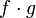$f \cdot g$ is also$n$ times differentiable at$x = x_0$, and we have: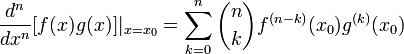$\frac{d^n}{dx^n}[f(x)g(x)]|_{x = x_0} = \sum_{k=0}^n \binom{n}{k} f^{(n - k)}(x_0)g^{(k)}(x_0)$
Here,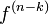$f^{(n - k)}$ denotes the$(n-k)^{th}$ derivative of$f$ (with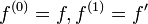$f^{(0)} = f, f^{(1)} = f'$, etc.),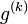$g^{(k)}$ denotes the$k^{th}$ derivative of$g$, and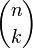$\binom{n}{k}$ is the binomial coefficient. These are the same as the coefficients that appear in the expansion of$\! (A + B)^n$.
generic point, named functions, point notation If$f$ and$g$ are functions of one variable, the following holds wherever the right side makes sense:$\! \frac{d^n}{dx^n}[f(x)g(x)] = \sum_{k=0}^n \binom{n}{k} f^{(n-k)}(x)g^{(k)}(x)$
generic point, named functions, point-free notation If$f$ and$g$ are functions of one variable, the following holds wherever the right side makes sense: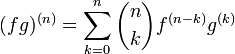$\! (fg)^{(n)} = \sum_{k=0}^n \binom{n}{k} f^{(n-k)}g^{(k)}$
Pure Leibniz notation Suppose$u$ and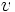$v$ are both variables functionally dependent on$x$. Then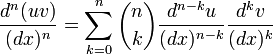$\frac{d^n(uv)}{(dx)^n} = \sum_{k=0}^n \binom{n}{k} \frac{d^{n-k}u}{(dx)^{n-k}}\frac{d^kv}{(dx)^k}$

### One-sided version

There are analogues of each of the statements with one-sided derivatives. Fill this in later

## Particular cases

Value of$n$ Formula for$\frac{d^n}{dx^n}[f(x)g(x)]$
1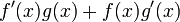$\! f'(x)g(x) + f(x)g'(x)$ (this is the usual product rule for differentiation).
2$\! f''(x)g(x) + 2f'(x)g'(x) + f(x)g''(x)$.
3$\! f'''(x)g(x) + 3f''(x)g'(x) + 3f'(x)g''(x) + g'''(x)$.
4$\! f^{(4)}(x)g(x) + 4f'''(x)g'(x) + 6f''(x)g''(x) + 4f'(x)g'''(x) + f(x)g^{(4)}(x)$
5$\! f^{(5)}(x)g(x) + 5f^{(4)}(x) g'(x) + 10f'''(x)g''(x) + 10f''(x)g'''(x) + 5f(x)g^{(4)}(x) + g^{(5)}(x)$

## Significance

### Qualitative and existential significance

Each of the versions has its own qualitative significance:

Version type Significance
specific point, named functions This tells us that if$f$ and$g$ are both$n$ times differentiable at a point$x_0$, so is$f \cdot g$.
generic point, named functions, point notation This tells us that if$f$ and$g$ are both$n$ times differentiable on an open interval, so is$f \cdot g$.
generic point, named functions, point-free notation This shows that the way that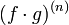$(f \cdot g)^{(n)}$ behaves is governed by the nature of the derivatives (up to the$n^{th}$) of$f$ and$g$. In particular, if$f^{(n)}$ and$g^{(n)}$ are both continuous functions on an interval, so is$(f \cdot g)^{(n)}$.

### Computational feasibility significance

Each of the versions has its own computational feasibility significance:

Version type Significance
specific point, named functions This tells us that knowing the values (in the sense of numerical values) of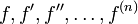$f,f',f'',\dots, f^{(n)}$ and$g,g',g'',\dots,g^{(n)}$ at a point$x_0$ allows us to compute the value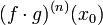$(f \cdot g)^{(n)}(x_0)$ by plugging into the formula and doing a bunch of multiplications and additions.
generic point, named functions This tells us that knowledge of the generic expressions for$f,f',f'',\dots, f^{(n)}$ and$g,g',g'',\dots,g^{(n)}$ allows us to compute the generic expression for$(f \cdot g)^{(n)}$.